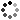# 6th Grade Math Writing Prompts

On Sale
\$4.00
\$4.00
Preview

Get your 6th grade math students writing with these thirty-five 6th grade math writing prompts that are aligned to the common core math standards. These questions go beyond basic fluency of math concepts. Questions help students analyze and think deeply about the mathematics. As a teacher, you can more easily assess understanding from well-structured questions where students must explain and give examples.

Correlating with the common core, these writing prompts contain the following:
• 6 Ratios and Proportions (RP)
• 6 Number Systems (NS)
• 7 Expressions and Equations (EE)
• 5 Geometry (G)
• 6 Statistics and Probability (SP)
• 5 Mathematical Practices (MP)
These writing prompts come in three different formats:
• Paper strips
• Half sheets of paper with space to respond
These can be used in a variety of ways in the classroom:
• Openers
• Exit slips
• Interactive notebooks
• Homework
• Assessments
• Games
• Centers
The questions are numbered to aid in the process of recording and grading.

Please feel free to contact me with any questions!

Michelle,
Make Sense of Math